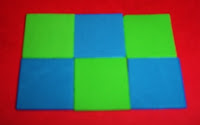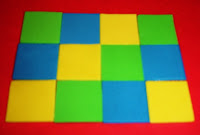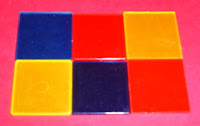## Saturday, February 4, 2012

### Exploring AREA with Manipulatives in Math

Using Manipulatives is beneficial when kids are first introduced to the Concept of AREA in math.

An easy inexpensive manipulative to make is little squares. I used foam bought at the dollar store but you could use construction paper, cardboard from cereal boxes, etc.

I used my paper cutter to cut the foam but you could use a scissors or a square paper punch. The squares can be any size as long as the squares are all the same size.

Children can start by making square or rectangular shapes and counting the small squares to find the AREA.Area = 6 square unitsArea = 12 square unitsArea = 16 square units

As children get older they can learn the FORMULA for the AREA of a RECTANGLE (length x width = area).

Here are a few other manipulatives that can be used (borrowed from other math manipulatives if you have them available).

The squares from a set of Attribute BlocksArea = 6 square units

The squares from sets of  TangramsArea = 4 square units

If you didn't see my previous post about using Cheez-Its, you can view it here.

Have fun with AREA!